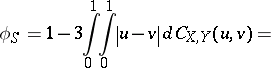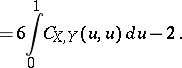Spearman rho metric

Spearman rho

The non-parametric correlation coefficient (or measure of association) known as Spearman's rho was first discussed by the psychologist C. Spearman in 1904 [a4] as a coefficient of correlation on ranks (cf. also Correlation coefficient; Rank statistic). In modern use, the term "correlation" refers to a measure of a linear relationship between variates (such as the Pearson product-moment correlation coefficient), while "measure of association" refers to a measure of a monotone relationship between variates (such as the Kendall tau metric and Spearman's rho). For an historical review of Spearman's rho and related coefficients, see [a2].

Spearman's rho, denoted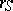, is computed by applying the Pearson product-moment correlation coefficient procedure to the ranks associated with a sample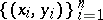. Let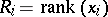and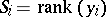; then computing the sample (Pearson) correlation coefficientfor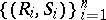yields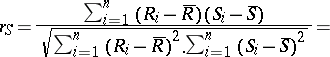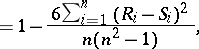where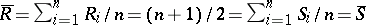. When ties exist in the data, the following adjusted formula foris used: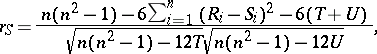whereforthe number ofobservations that are tied at a given rank, and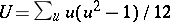forthe number ofobservations that are tied at a given rank. For details on the use ofin hypothesis testing, and for large-sample theory, see [a1].

Ifandare random variables (cf. Random variable) with respective distribution functionsand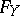, then the population parameter estimated by, usually denoted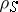, is defined to be the Pearson product-moment correlation coefficient of the random variables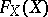and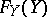: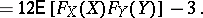Spearman'sis occasionally referred to as the grade correlation coefficient, sinceandare sometimes called the "grades" ofand.

Like Kendall's tau,is a measure of association based on the notion of concordance. One says that two pairsand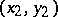of real numbers are concordant ifandor ifand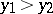(i.e., if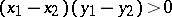); and discordant if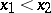andor if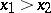and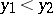(i.e., if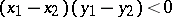). Now, let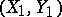,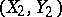and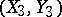be independent random vectors with the same distribution as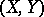. Then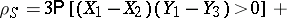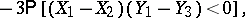that is,is proportional to the difference between the probabilities of concordance and discordance between the random vectorsand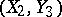(clearly,can be replaced by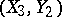).

Whenandare continuous,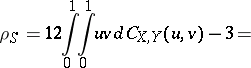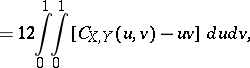where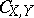is the copula ofand. Consequently,is invariant under strictly increasing transformations ofand, a propertyshares with Kendall's tau but not with the Pearson product-moment correlation coefficient. Note thatis proportional to the signed volume between the graphs of the copula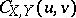and the "product" copula, the copula of independent random variables. For a survey of copulas and their relationship with measures of association, see [a3].

Spearman [a5] also proposed an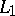version of, known as Spearman's footrule, based on absolute differences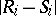in ranks rather than squared differences:The population parameterestimated byis given by